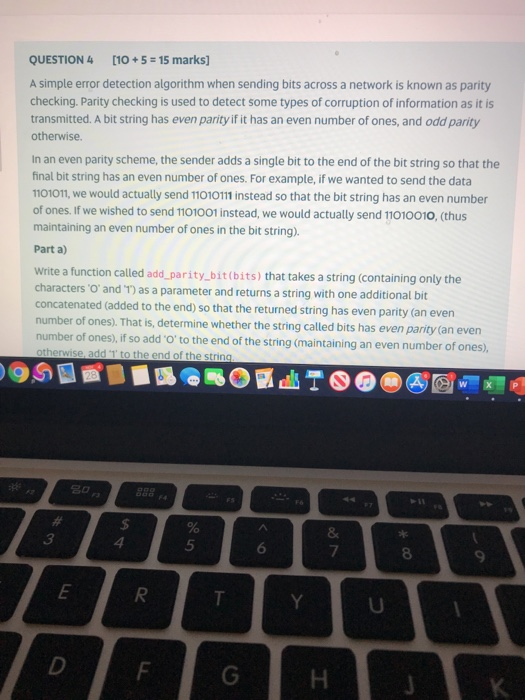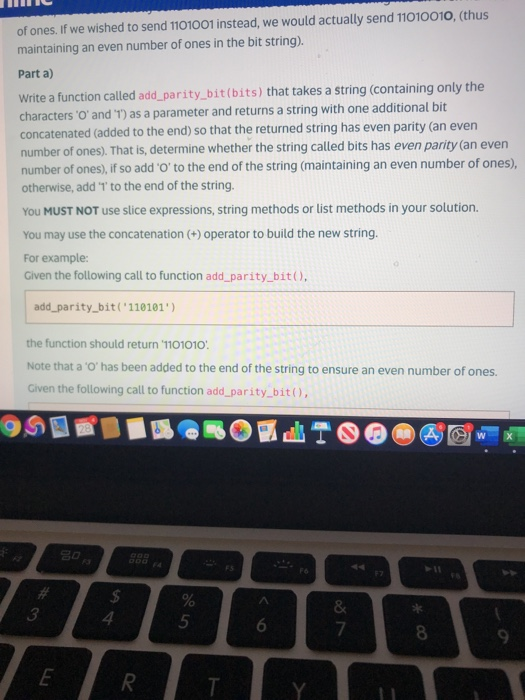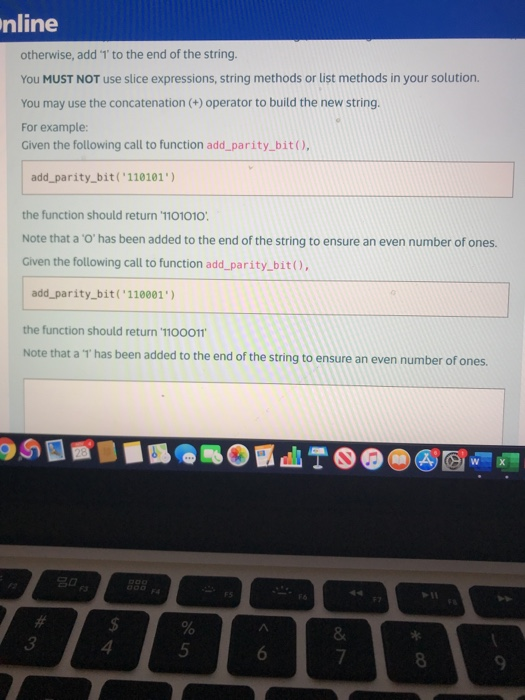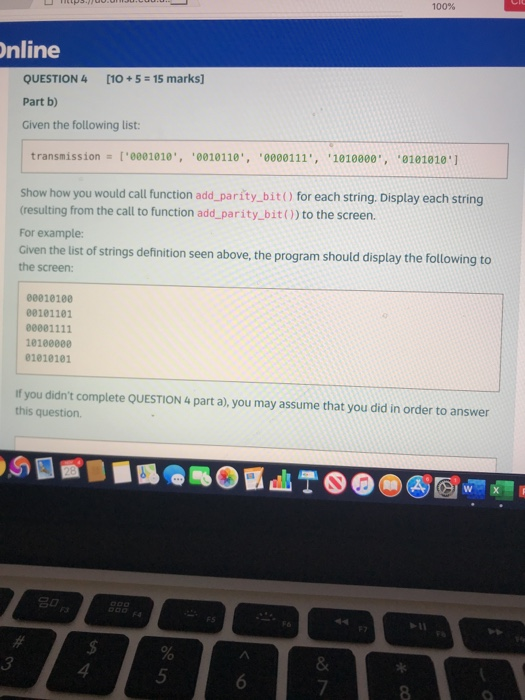# (Solved) : Question 4 10 5 15 Marks Simple Error Detection Algorithm Sending Bits Across Network Know Q42789171 . . .

code in pythonQUESTION 4 [10+ 5 = 15 marks) A simple error detection algorithm when sending bits across a network is known as parity checking. Parity checking is used to detect some types of corruption of information as it is transmitted. A bit string has even parity if it has an even number of ones, and odd parity otherwise In an even parity scheme, the sender adds a single bit to the end of the bit string so that the final bit string has an even number of ones. For example, if we wanted to send the data 1101011, we would actually send 11010111 instead so that the bit string has an even number of ones. If we wished to send 1101001 instead, we would actually send 11010010, (thus maintaining an even number of ones in the bit string). Parta) Write a function called add_parity_bitbits) that takes a string (containing only the characters ‘O’ and ‘1) as a parameter and returns a string with one additional bit concatenated (added to the end) so that the returned string has even parity (an even number of ones). That is, determine whether the string called bits has even parity (an even number of ones), if so add ‘O’ to the end of the string (maintaining an even number of ones), otherwise, add ‘T’ to the end of the string TILI of ones. If we wished to send 1101001 instead, we would actually send 11010010. (thus maintaining an even number of ones in the bit string). Parta) Write a function called add_parity_bit(bits) that takes a string (containing only the characters ‘O’ and ‘1’) as a parameter and returns a string with one additional bit concatenated (added to the end) so that the returned string has even parity (an even number of ones). That is, determine whether the string called bits has even parity (an even number of ones), if so add ‘O’ to the end of the string (maintaining an even number of ones), otherwise, add ‘T to the end of the string. You MUST NOT use slice expressions, string methods or list methods in your solution. You may use the concatenation (+) operator to build the new string. For example: Given the following call to function add_parity_bit(), add_parity_bit(‘110101’) the function should return 1101010 Note that a ‘O’ has been added to the end of the string to ensure an even number of ones. Given the following call to function add_parity_bit ģ тү | nline otherwise, add 1 to the end of the string. You MUST NOT use slice expressions, string methods or list methods in your solution. You may use the concatenation (+) operator to build the new string. For example: Given the following call to function add_parity_bito, add_parity_bit(‘110101’) the function should return 1101010 Note that a ‘O’ has been added to the end of the string to ensure an even number of ones. Given the following call to function add_parity_bito, add_parity_bit(‘110001′) the function should return 1100011’ Note that a ‘T has been added to the end string to ensure an even number of ones. 3 FL5 00. U .L .U . 100% Online QUESTION 4 [10+ 5 = 15 marks] Part b) Given the following list: transmission = 1’0001010’, ‘0010110’, ‘0000111’, ‘1010000’, *01010101 Show how you would call function add_parity_bit for each string. Display each string (resulting from the call to function add_parity_bit() to the screen. For example: Given the list of strings definition seen above, the program should display the following to the screen: 00010100 00101101 00001111 10100000 01010101 If you didn’t complete QUESTION 4 part a), you may assume that you did in order to answer this question gors ģ Show transcribed image text QUESTION 4 [10+ 5 = 15 marks) A simple error detection algorithm when sending bits across a network is known as parity checking. Parity checking is used to detect some types of corruption of information as it is transmitted. A bit string has even parity if it has an even number of ones, and odd parity otherwise In an even parity scheme, the sender adds a single bit to the end of the bit string so that the final bit string has an even number of ones. For example, if we wanted to send the data 1101011, we would actually send 11010111 instead so that the bit string has an even number of ones. If we wished to send 1101001 instead, we would actually send 11010010, (thus maintaining an even number of ones in the bit string). Parta) Write a function called add_parity_bitbits) that takes a string (containing only the characters ‘O’ and ‘1) as a parameter and returns a string with one additional bit concatenated (added to the end) so that the returned string has even parity (an even number of ones). That is, determine whether the string called bits has even parity (an even number of ones), if so add ‘O’ to the end of the string (maintaining an even number of ones), otherwise, add ‘T’ to the end of the string
nline otherwise, add 1 to the end of the string. You MUST NOT use slice expressions, string methods or list methods in your solution. You may use the concatenation (+) operator to build the new string. For example: Given the following call to function add_parity_bito, add_parity_bit(‘110101’) the function should return 1101010 Note that a ‘O’ has been added to the end of the string to ensure an even number of ones. Given the following call to function add_parity_bito, add_parity_bit(‘110001′) the function should return 1100011’ Note that a ‘T has been added to the end string to ensure an even number of ones. 3
FL5 00. U .L .U . 100% Online QUESTION 4 [10+ 5 = 15 marks] Part b) Given the following list: transmission = 1’0001010′, ‘0010110’, ‘0000111’, ‘1010000’, *01010101 Show how you would call function add_parity_bit for each string. Display each string (resulting from the call to function add_parity_bit() to the screen. For example: Given the list of strings definition seen above, the program should display the following to the screen: 00010100 00101101 00001111 10100000 01010101 If you didn’t complete QUESTION 4 part a), you may assume that you did in order to answer this question gors ģ

Answer to QUESTION 4 [10+ 5 = 15 marks) A simple error detection algorithm when sending bits across a network is known as parity c…

We are the best freelance writing portal. Looking for online writing, editing or proofreading jobs? We have plenty of writing assignments to handle.